0
130

# Paper Cutting and Folding Questions for SSC

Download SSC CGL Questions on Paper Cutting and Folding PDF based on previous papers very useful for SSC CGL exams. Paper Cutting and Folding Questions for SSC exams.

Instructions

From the given answer figures, select the one in which the question figure is hidden/embedded.

Question 1: A piece of paper is folded and cut as shown below in the question figures. From the given answer figures, indicate how it will appear when opened. Questions Figure :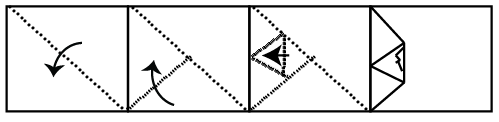a)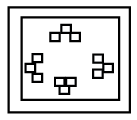b)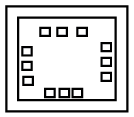c)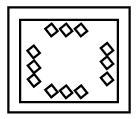d)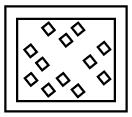Question 2: A piece of paper is folded and cut as shown below in the question figures. From the given answer figures, indicates how it will appear when opened. Questions Figure :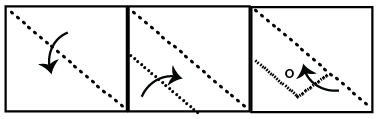a)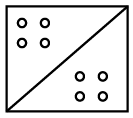b)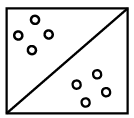c)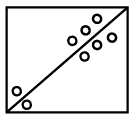d)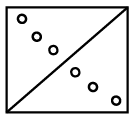Question 3: A piece of paper is folded and punched as shown below in the question figures. From the given answer figures, indicate how. it will appear when opened.
Question Figure :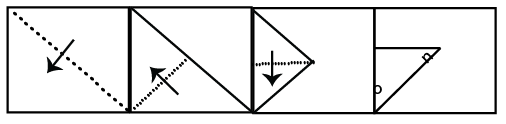a)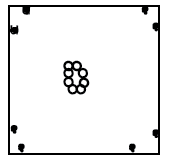b)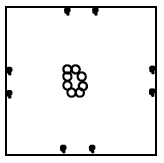c)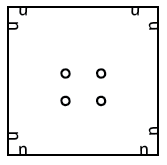d)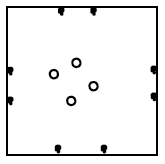Question 4: A piece of paper is folded and punched as shown below in the question figures.
From the given answer figures, indicate how it will appear when opened.
Question Figure :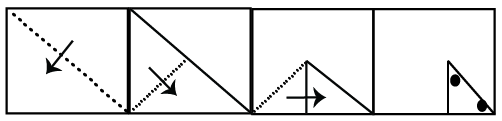a)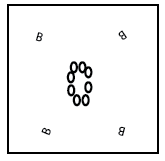b)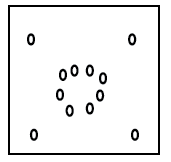c)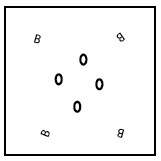d)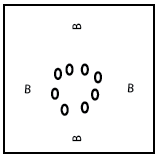Question 5: A piece of papers is folded and punched as shown below in the question figures. From the given answer figures, indicate how it will appear when opened.

a)

b)

c)

d)

Question 6: A piece of paper is folded and punched as shown below in the question figures. From the given answer figures, indicates how it will appear when opened.

a)

b)

c)

d)

Question 7: A piece of paper is folded and punched as shown below in the question figures. From the given answer figures, indicate how it will appear when opened?

a)

b)

c)

d) Figure 4

Question 8: A piece of paper is folded and punched as shown in the below question figures. From the given answer figures, indicate how it will appear when opened.

a)

b)

c)

d)

Question 9: A piece of paper is folded and punched as shown below in the question figures. From the answer figures, indicate how it will appear?

a)

b)

c)

d)

Question 10: A piece of paper is folded and punched as shown below in the question figures. From the given answer figures, indicate how it will appear when opened.

a)

b)

c)

d)